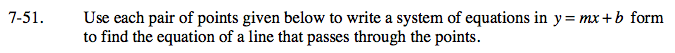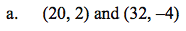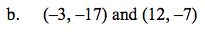Home > A2C > Chapter 7 > Lesson 7.1.4 > Problem7-51

7-51.
1. Use each pair of points given below to write a system of equations in y = mx + b form to find the equation of a line that passes through the points. 7-51 HW eTool (Desmos). Homework Help ✎

1. (20, 2) and (32, −4)

2. (−3, −17) and (12, −7)20 = m(2) + b

32 = m(−4) + bRefer to part (a).

Use the eTool below to explore the graph.
Click the link at right for the full version of the eTool: A2C 7-51 HW eTool